# Resource 4: Examples of Physics in action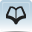Teacher resource for planning or adapting to use with pupils

## Places to visit and examples you might see

 Place Examples Physics principles they use Garage or workshop Hydraulic jacks Pressure = force/area and pressure is transmitted through a fluid (oil), so input pressure = output pressureUsed as a ‘force multiplier’ Brace, screwdriver Input force x input distance from axle = output force x output distance A force multiplier Building site Pulleys Input force x distance it moves = output load raised x height it is lifted.A force multiplier Wheelbarrows and levers Input force x input distance from wheel axle or pivot = output force x output distance from pivotA force multiplier Kitchen or bakery Can openers, potato chippers, nut-cracker Examples of levers as force multipliers, so small force exerted by operator on the handle produces a large force on the object:Input force x input distance from pivot = output force x output distance Knives and skewers Narrow blade edges and fine points cut into the material more easily because, for the same force, reducing the area increases the pressure Playground Seesaw Another lever example: a lighter person sits further out to balance a heavier person.Clockwise moment = anticlockwise moment Farm/garden Shears/secateurs/ branch cutters Force multipliers:Input force x input distance from pivot = output force x output distance

## Some examples of force multipliers

Hydraulic jack:

You use a small force but push further to raise the large load a smaller distance.

Input pressure = output pressure because the pressure is transmitted by oil.

• narrow input piston cylinder with area A1 , small input force F1
• wider output piston cylinder with area A2, larger output force F2
• force on output piston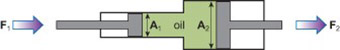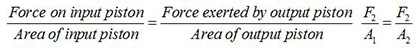Levers , e.g. see-saw:

Clockwise turning force x distance from pivot = anticlockwise turning force x distance from pivot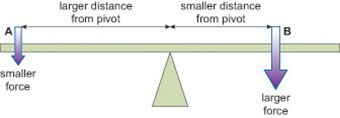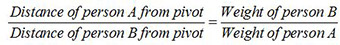Cutting tools e.g. secateurs, shears: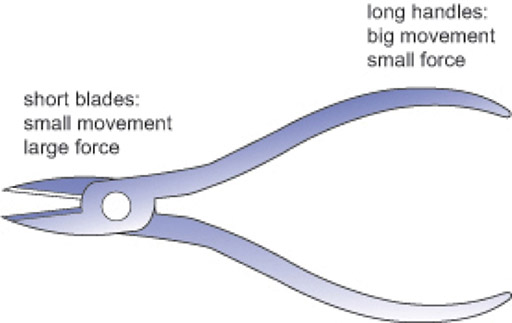Resource 3: Everyday examples of pressure

Resource 5: Keeping things cool